Next: Damped Oscillatory Motion Up: One-Dimensional Motion Previous: Velocity Dependent Forces

Simple Harmonic Motion

Consider the motion of a point particle of mass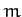which is slightly displaced from a stable equilibrium point at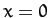. Suppose that the particle is moving in the conservative force-field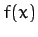. According to the analysis of Section 3.2, in order forto be a stable equilibrium point we require both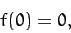(72)

and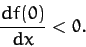(73)

Now, our particle obeys Newton's second law of motion,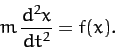(74)

Let us assume that it always stays fairly close to its equilibrium point. In this case, to a good approximation, we can representvia a truncated Taylor expansion about this point. In other words,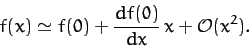(75)

However, according to (72) and (73), the above expression can be written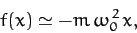(76)

where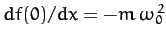. Hence, we conclude that our particle satisfies the following approximate equation of motion,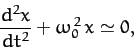(77)

provided that it does not stray too far from its equilibrium point: i.e., provided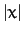does not become too large.

Equation (77) is called the simple harmonic equation, and governs the motion of all one-dimensional conservative systems which are slightly perturbed from some stable equilibrium state. The solution of Equation (77) is well-known: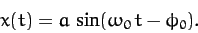(78)

The pattern of motion described by above expression, which is called simple harmonic motion, is periodic in time, with repetition period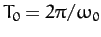, and oscillates between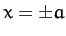. Here,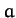is called the amplitude of the motion. The parameter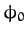, known as the phase angle, simply shifts the pattern of motion backward and forward in time. Figure 4 shows some examples of simple harmonic motion. Here,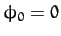,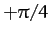, and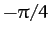correspond to the solid, short-dashed, and long-dashed curves, respectively.

Note that the frequency,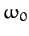--and, hence, the period,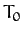--of simple harmonic motion is determined by the parameters appearing in the simple harmonic equation, (77). However, the amplitude,, and the phase angle,, are the two integration constants of this second-order ordinary differential equation, and are, thus, determined by the initial conditions: i.e., by the particle's initial displacement and velocity.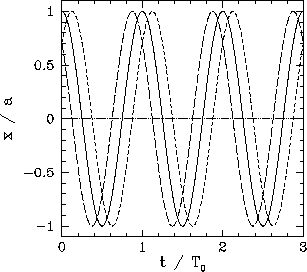Now, from Equations (45) and (76), the potential energy of our particle at positionis approximately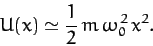(79)

Hence, the total energy is written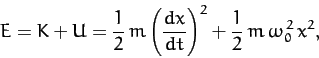(80)

giving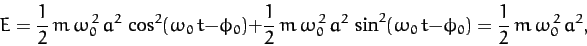(81)

where use has been made of Equation (78), and the trigonometric identity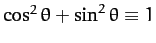. Note that the total energy is constant in time, as is to be expected for a conservative system, and is proportional to the amplitude squared of the motion.Next: Damped Oscillatory Motion Up: One-Dimensional Motion Previous: Velocity Dependent Forces
Richard Fitzpatrick 2011-03-31